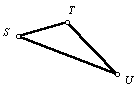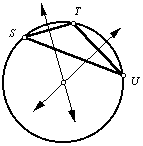Given a triangle, circumscribe a circle.

 1. Begin with triangle STU.2. If a circle is circumscribed around the triangle, then all three vertices will be points on the circle, so follow the instructions for construction of a circle through three given points.Back to Geometry Construction Reference